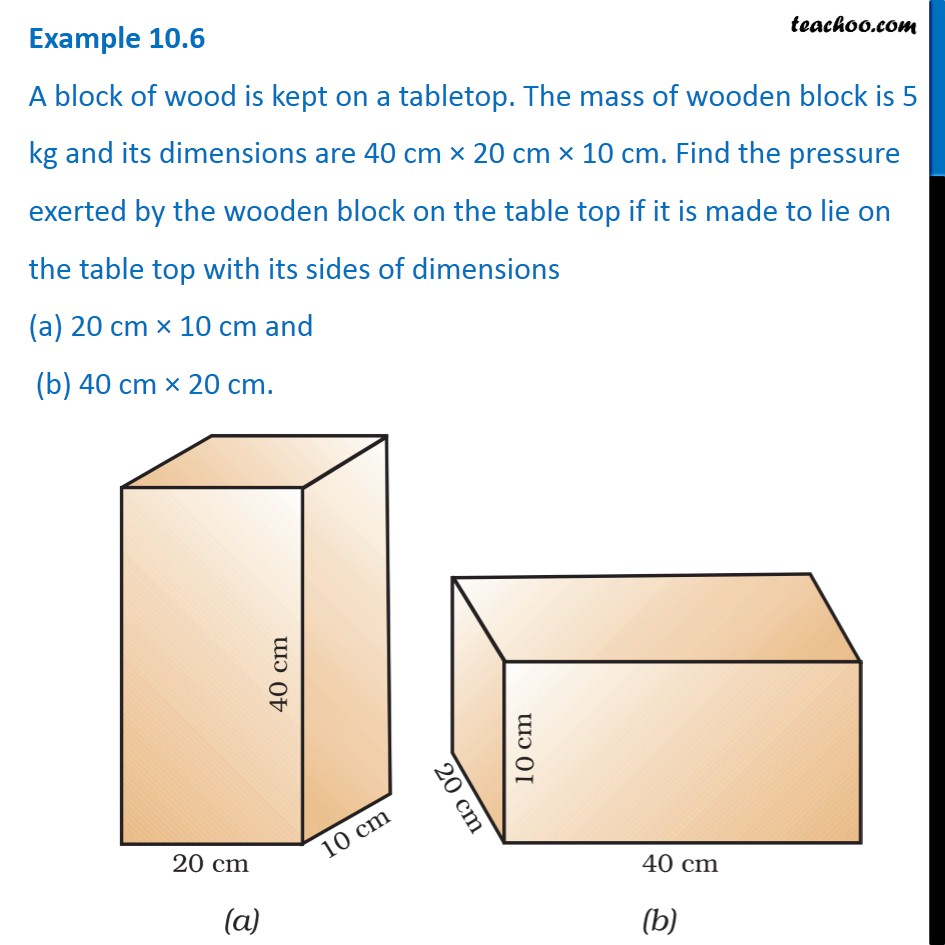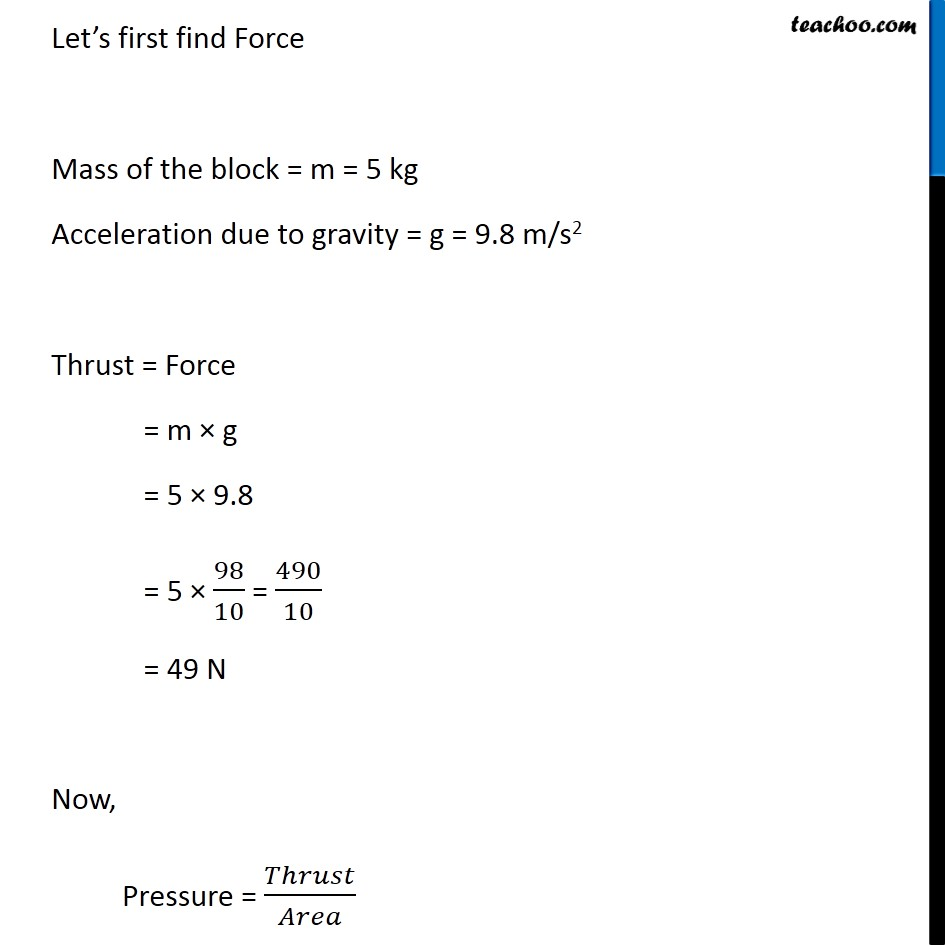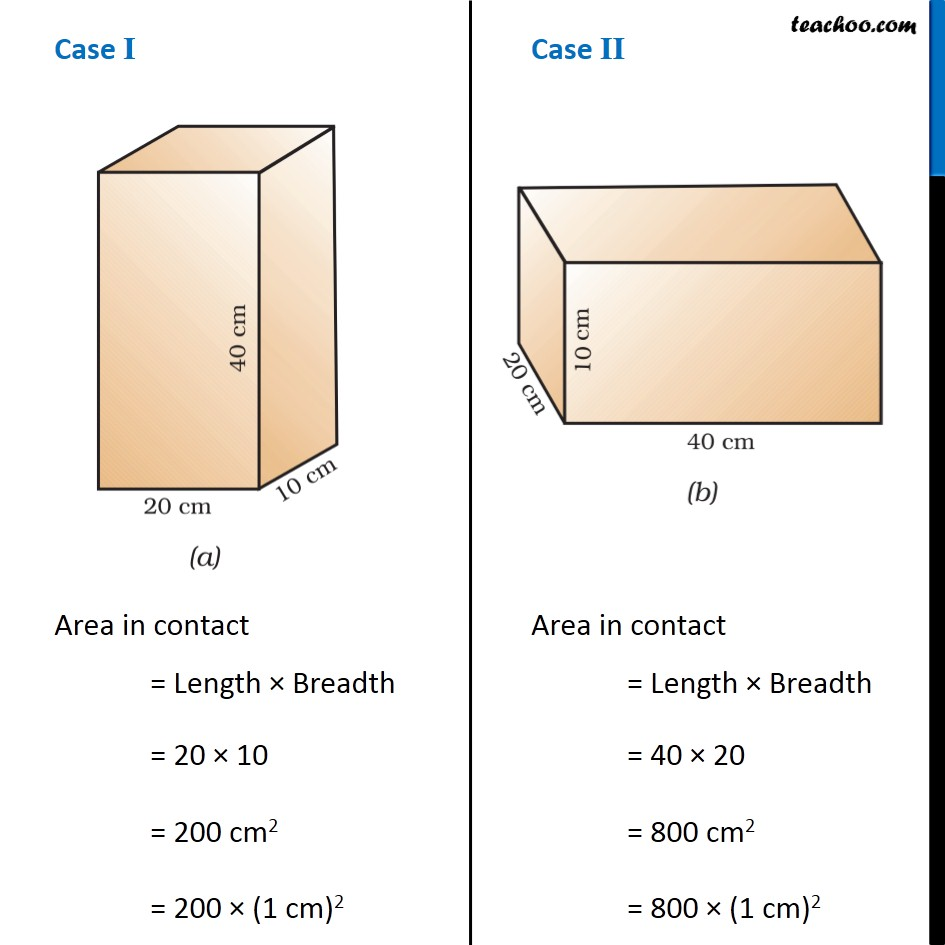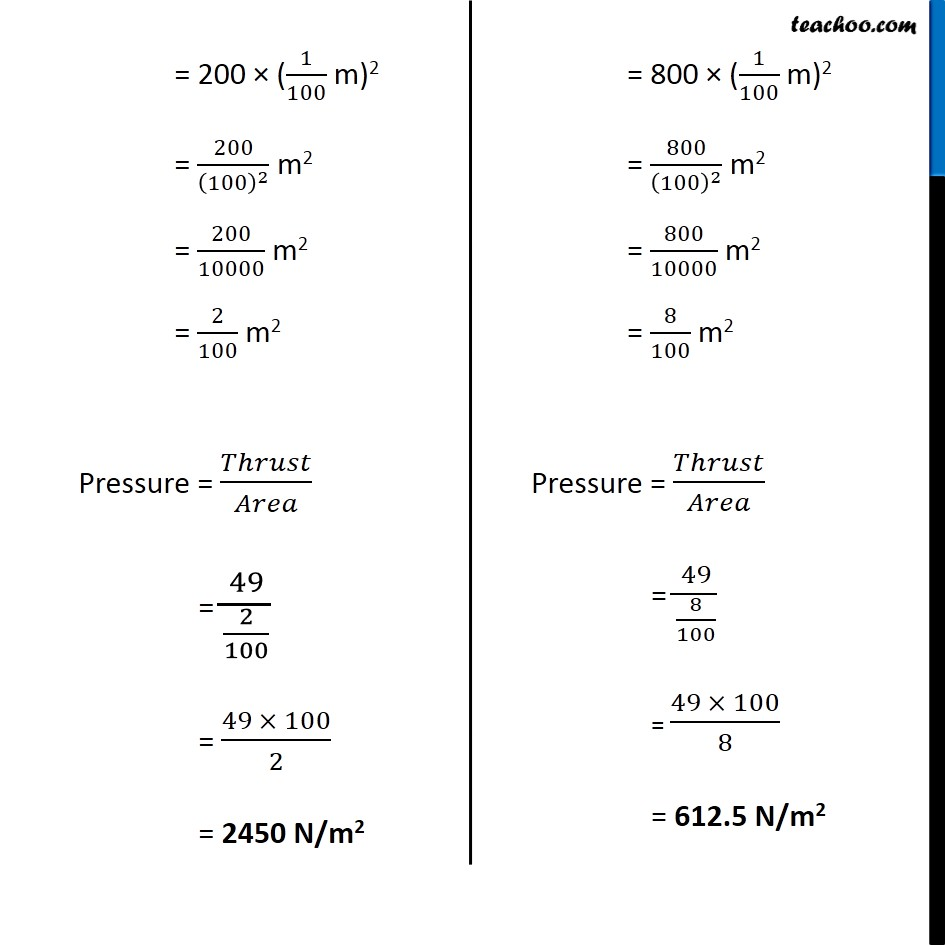Examples from NCERT Book

Class 9
Chapter 10 Class 9 - GravitationLearn in your speed, with individual attention - Teachoo Maths 1-on-1 Class

### Transcript

Example 10.6 A block of wood is kept on a tabletop. The mass of wooden block is 5 kg and its dimensions are 40 cm × 20 cm × 10 cm. Find the pressure exerted by the wooden block on the table top if it is made to lie on the table top with its sides of dimensions (a) 20 cm × 10 cm and (b) 40 cm × 20 cm. Let’s first find Force Mass of the block = m = 5 kg Acceleration due to gravity = g = 9.8 m/s2 Thrust = Force = m × g = 5 × 9.8 = 5 × 98/10 = 490/10 = 49 N Now, Pressure = 𝑇ℎ𝑟𝑢𝑠𝑡/𝐴𝑟𝑒𝑎 Case I Area in contact = Length × Breadth = 20 × 10 = 200 cm2 = 200 × (1 cm)2 Case II Area in contact = Length × Breadth = 40 × 20 = 800 cm2 = 800 × (1 cm)2 = 200 × (1/100 m)2 = 200/(100)^2 m2 = 200/10000 m2 = 2/100 m2 Pressure = 𝑇ℎ𝑟𝑢𝑠𝑡/𝐴𝑟𝑒𝑎 = 49/(2/100) = (49 × 100)/2 = 2450 N/m2 = 800 × (1/100 m)2 = 800/(100)^2 m2 = 800/10000 m2 = 8/100 m2 Pressure = 𝑇ℎ𝑟𝑢𝑠𝑡/𝐴𝑟𝑒𝑎 = 49/(8/100) = (49 × 100)/8 = 612.5 N/m2